# Resources tagged with: Scalar products

Filter by: Content type:
Age range:
Challenge level:

### There are 11 results

Broad Topics > Vectors and Matrices > Scalar products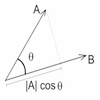### Cross with the Scalar Product

##### Age 16 to 18 Challenge Level:

Explore the meaning of the scalar and vector cross products and see how the two are related.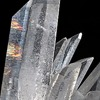### Coordinated Crystals

##### Age 16 to 18 Challenge Level:

Explore the lattice and vector structure of this crystal.##### Age 16 to 18 Challenge Level:

A quadrilateral changes shape with the edge lengths constant. Show the scalar product of the diagonals is constant. If the diagonals are perpendicular in one position are they always perpendicular?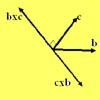### Multiplication of Vectors

##### Age 16 to 18

An account of multiplication of vectors, both scalar products and vector products.### Air Routes

##### Age 16 to 18 Challenge Level:

Find the distance of the shortest air route at an altitude of 6000 metres between London and Cape Town given the latitudes and longitudes. A simple application of scalar products of vectors.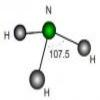### Bond Angles

##### Age 16 to 18 Challenge Level:

Think about the bond angles occurring in a simple tetrahedral molecule and ammonia.### Quaternions and Reflections

##### Age 16 to 18 Challenge Level:

See how 4 dimensional quaternions involve vectors in 3-space and how the quaternion function F(v) = nvn gives a simple algebraic method of working with reflections in planes in 3-space.### Cubestick

##### Age 16 to 18 Challenge Level:

Stick some cubes together to make a cuboid. Find two of the angles by as many different methods as you can devise.##### Age 16 to 18 Challenge Level:

As a quadrilateral Q is deformed (keeping the edge lengths constnt) the diagonals and the angle X between them change. Prove that the area of Q is proportional to tanX.### Pythagoras on a Sphere

##### Age 16 to 18 Challenge Level:

Prove Pythagoras' Theorem for right-angled spherical triangles.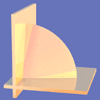### Walls

##### Age 16 to 18 Challenge Level:

Plane 1 contains points A, B and C and plane 2 contains points A and B. Find all the points on plane 2 such that the two planes are perpendicular.